### Choose language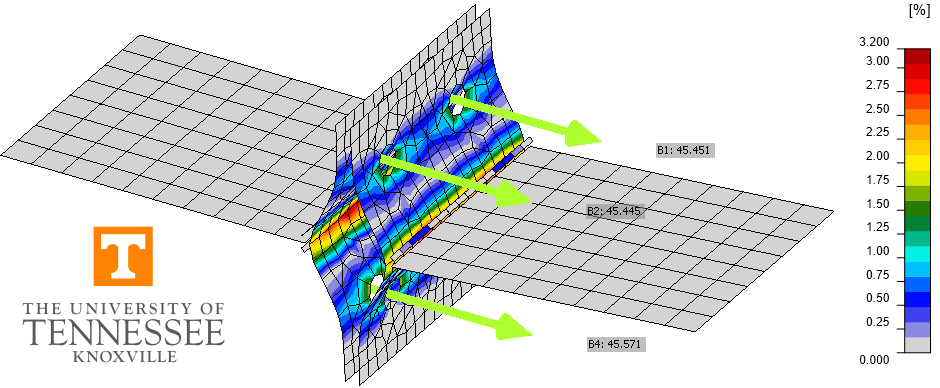# T-stub connections (AISC)

This example is part of a series that compares IDEA StatiCa to traditional calculations for US practice. Prying action and the strength of bolts subjected to combined tension and shear are highlighted through the examination of T-stub connections.
$$This verification example was prepared by Mark D. Denavit and Kayla Truman-Jarrell in a joint project of The University of Tennessee and IDEA StatiCa.DescriptionA comparison between results from the component-based finite element method (CBFEM) and traditional calculation methods used in US practice for T-stub connections is presented in this study. A schematic of the connection investigated is presented in Fig. 1. The limit states evaluated are slip, bolt tension and shear interaction strength, and flexural yielding of the flanges of the T-stub and the beam. The impact of prying action is considered.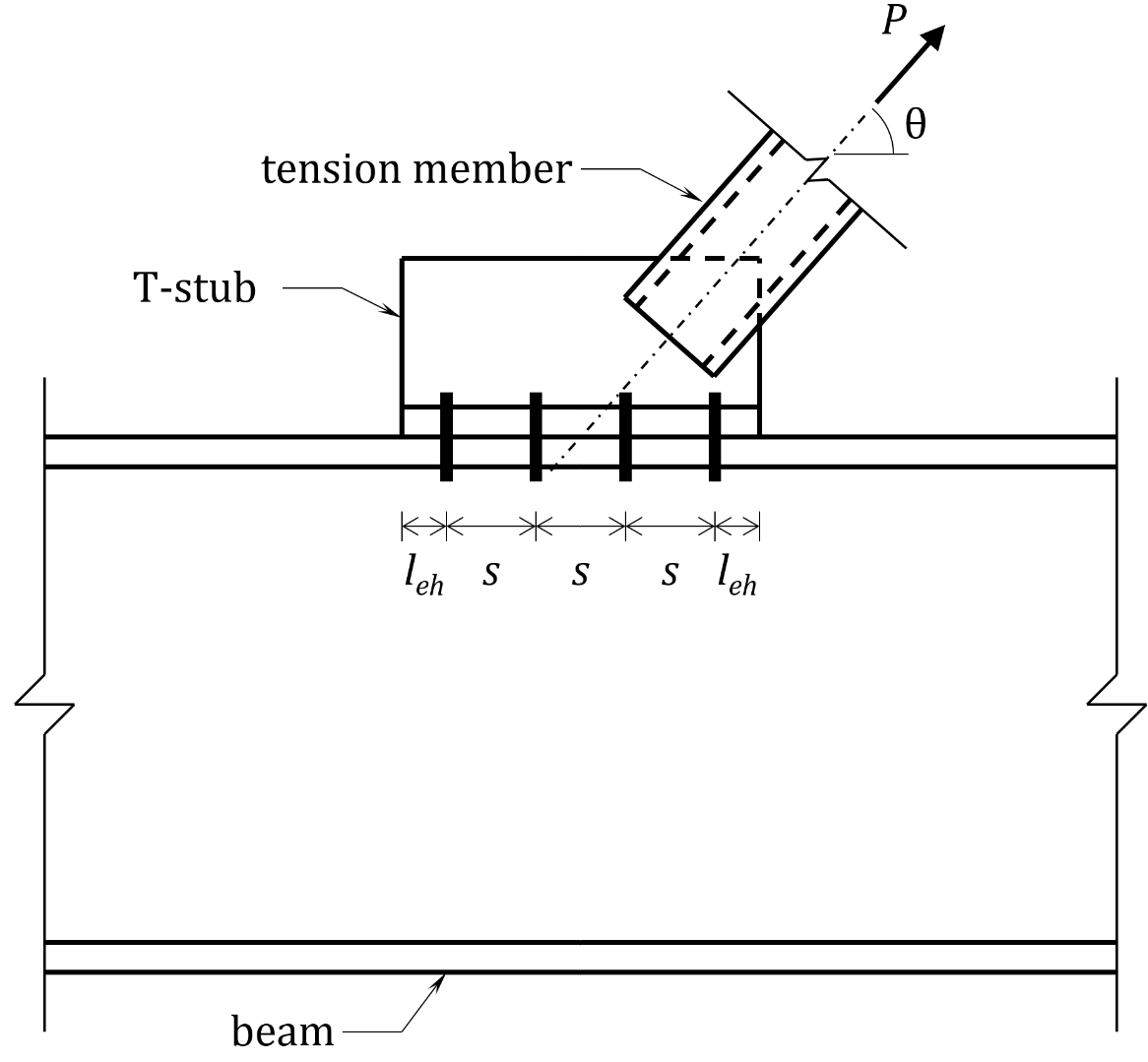Fig. 1 Schematic of the T-stub connection investigated in this studyFor all connections investigated, the beam is a wide flange conforming to ASTM A992 (Fy = 50 ksi and Fu = 65 ksi) and the T-stub is built up from plates conforming to ASTM A572 Gr. 50 (Fy = 50 ksi and Fu = 65 ksi). Butt welds are used between the stem and the flange of the T-stub and between the tension member and the stem of the T-stub to simplify the evaluation. Each connection investigated had (8) 3/4 in. diameter bolts (i.e., 2 rows of 4 bolts) in standard holes with spacing, s = 3 in., edge distance leh = 1.5 in., and gage, g = 5.5 in.The traditional calculations were performed in accordance with the provisions for load and resistance factor design (LRFD) in the AISC Specification (2016) with prying action considered as described in Part 9 of the AISC Manual (2017).The CBFEM results were obtained from IDEA StatiCa version 21.0. The maximum permitted loads were determined iteratively by adjusting the applied load input to a value that the program deems safe but if increased by a small amount (0.1 kip) the program would deem unsafe. DR type analyses can help identify maximum permitted loads. However, some approximation is made in the evaluation of the joint design resistance, therefore all results in this report are based on EPS type analyses.Slip-critical connectionsThe first limit state investigated is slip. The configuration of this example matches that of Example J.5 of the AISC Design Examples v15.1 (AISC, 2019). Additional details of the connection include that the bolts are Group A (e.g. A325) with threads not excluded from the shear planes; the beam is a W18×175; the T-stub web thickness was tw = 0.75 in.; the T-stub flange width was bf = 8.0 in.; the T-stub flange thickness varied; and θ = 53.1°. A three-dimensional view of one of the connections investigated is presented in Fig. 2.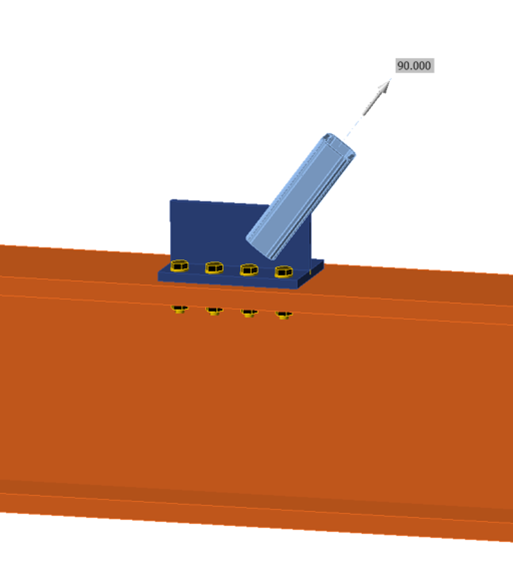Fig. 2 Three-dimension view of the connection investigated.Calculations were performed for five T-stub flange thicknesses between 0.5 in. and 1.5 in. The maximum factored tensile load which could be applied to the connection is presented in Fig. 3. For the traditional calculations, the maximum load does not vary with T-stub flange thickness except for the thinnest T-stub flange thickness where a slight reduction in maximum load is observed. Slip is the controlling limit state for all but the thinnest T-stub flange thickness which is controlled by the tensile strength of the bolts and flexural yielding of the T-stub. For the CBFEM results, the maximum load varies continuously with T-stub flange thickness.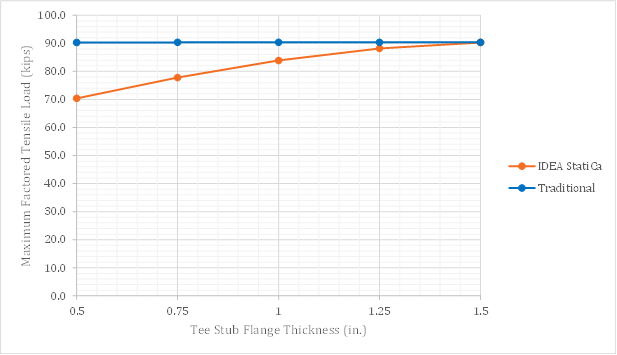Fig. 3 Design strength vs. T-stub flange thickness for slip-critical connectionsThe reason for the discrepancy can be identified through an examination of the detailed results provided by IDEA StatiCa. For this slip-critical connection, which is subjected to tension and shear, the provisions of Section J3.9 of the AISC Specification (2016) apply. Specifically, a reduction factor, ksc, which depends on the required tension force, is applied to the slip resistance. IDEA StatiCa includes the prying force in the required tension force used to compute ksc. This is conservative as it is not required by the AISC Specification (2016) and because prying forces do not reduce the clamping force which provides the slip resistance. For the thinnest flange investigated, IDEA StatiCa results in 23% less strength than the traditional calculations. For the thickest flange investigated, where prying action is prevented, IDEA StatiCa and the traditional calculations result in the same strength.Prying actionPrying action impacts the evaluation of plate bending strength and bolt strength. Part 9 of the AISC Manual (2017) presents equations that account for prying action. The equations are presented in several forms for various design situations. In this work, plate bending is evaluated by comparing the thickness of the component (i.e., T-stub flange or beam flange) to tmin as given by Equation 9-19 of the AISC Manual and bolt strength is evaluated by comparing the required bolt strength (i.e., Psinθ divided by the number of bolts) to the available tensile strength including the effects of prying action Tc as given by Equation 9-27 of the AISC Manual. Noting that LRFD was used for these analyses, tmin is calculated as:$t_{min} = \sqrt{\frac{4T_ub'}{\phi p F_u (1+\delta \alpha ') }}$$b'= b-\frac{d_b}{2}$$\delta = 1 - \frac{d'}{p}$$\beta = \frac{1}{\rho} \left ( \frac{B_c}{T_u}-1 \right )$$\rho = \frac{b'}{a'}$$a' = \left ( a + \frac{d_b}{2} \right ) \le \left ( 1.25 b + \frac{d_b}{2} \right )$If β ≥ 1$\alpha ' = 1$If β < 1$\alpha ' = \textrm{min} \left ( 1, \, \frac{1}{\delta} \frac{\beta}{1-\beta} \right )$where, Bc = available tension per bolt based on the limit state of tension or the combined limit states of tension and shear rupture = ϕrn Fu = specified minimum tensile strength of the connecting element Tu = required tension force per bolt using LRFD load combinations = Psinθ/nb a = distance from bolt centerline to the edge of the fitting b = the distance from bolt centerline to the face of the T-stem db = bolt diameter d' = hole diameter p = tributary length, based on yield line theory ϕ = 0.9 (for plate bending) Noting that LRFD was used for these analyses, Tc is calculated as:$T_c = B_c Q$If $$\alpha ' < 0$$ (indicating the fitting has sufficient strength and stiffness).$Q=1$If $$0 \le \alpha ' \le 1$$ (indicating sufficient strength to develop full bolt available tension strength, but insufficient to prevent prying)$Q = \left ( \frac{t}{t_c} \right )^2 (1+\delta \alpha ' )$If $$\alpha ' > 1$$ (indicating insufficient strength to develop full bolt tensile strength)$Q= \left ( \frac{t}{t_c} \right )^2 (1+\delta)$Note that the equation for for the determination of Q is different than the one used for the determination of tmin.$\alpha ' = \frac{1}{\delta (1+ \rho)} \left [ \left ( \frac{t_c}{t} \right )^2-1 \right ]$$t_c = \sqrt{\frac{4B_c b'}{\phi p F_u}}$where, t = thickness of the component Prying of the T-stubThe second investigation examines the strength of the T-stub and bolts. Same as the previous investigation, the bolts are Group A (e.g. A325) with threads not excluded from the shear planes; the beam is a W18×175; the T-stub web thickness was tw = 0.75 in.; the T-stub flange width was bf = 8.0 in.; the T-stub flange thickness varied; and θ = 53.1°. Different from the previous investigation, the connections were not slip-critical. Calculations were performed for eight T-stub flange thicknesses between 0.25 in. and 1.25 in. The maximum factored tensile load which could be applied to the connection is presented in Fig. 4. As expected, for both the traditional calculation results and the IDEA StatiCa results, the maximum factored tensile load increases with T-stub flange thickness until a plateau is reached where prying action is prevented. At the plateau, the strength of the connection is governed by the provisions of Section J3.7 of the AISC Specification (2016) and results from the traditional calculations and IDEA StatiCa match. Where prying action impacts the strength of the connection, there are differences between the traditional calculations which follow the guidance of Part 9 of the AISC Manual (2017) and IDEA StatiCa which explicitly models the connection using the CBFEM.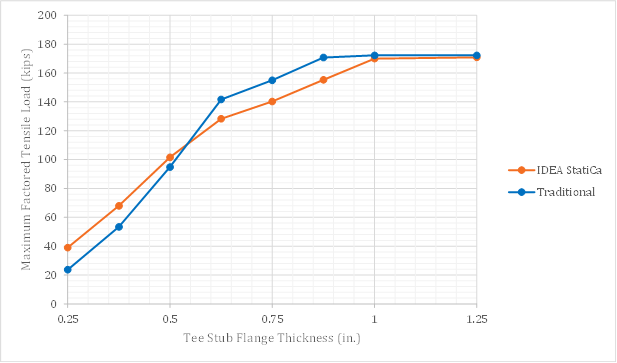Fig. 4 Design strength vs. T-stub flange thickness for bearing-type connectionsTypically, available flexural strength is computed based on the yield strength, Fy. The equations for prying action presented in Part 9 of the AISC Manual (2017) are based on the tensile strength, Fu, noting that using Fu in lieu of Fy provides a better correlation with available test data. Fig. 5 presents the same data as Fig. 4 but with the addition of traditional calculations using Fy in lieu of Fu. For T-stub flange thickness of 3/4 in. and 7/8 in., the use of Fy in the traditional calculation brings the strength closer to IDEA StatiCa (where strength is also based on Fy). For greater thicknesses, the bolt strength controls, so the choice of Fy or Fu does not impact the results. For smaller thicknesses, the use of Fy in the traditional calculations increases the discrepancy.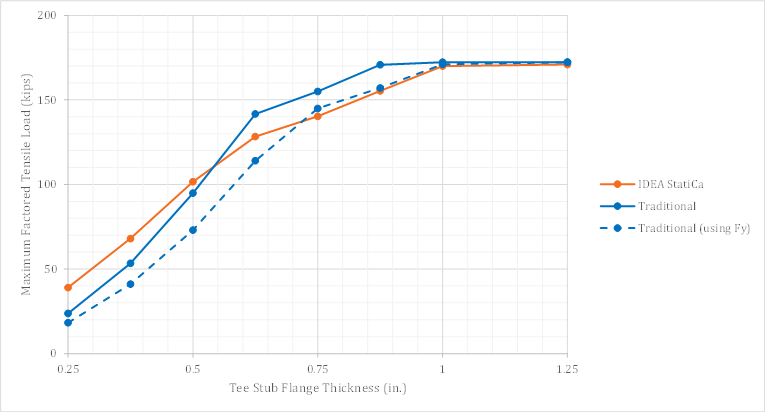Fig. 5 Design strength vs. T-stub flange thickness for bearing-type connections – including comparison to traditional calculations using F­yThe discrepancy between results from traditional calculations and IDEA StatiCa for prying action of thinner plates has been observed and investigated previously. Wald et al. (2020) compared traditional calculations to results of the component-based finite element method and to results from a research finite element model. The results showed that while the component-based finite element method does result in greater strength than the traditional calculations for thinner plates, there remains a significant margin of safety when compared to the research model. The study by Wald et al. (2020) was expanded in this work by adding a comparison to the strength computed using the equations for prying action presented in Part 9 of the AISC Manual (2017). Results, which are superimposed on existing results from Fig. 5.1.5 from Wald et al. (2020), are presented in Fig. 6. For thinner plates, the AISC results are close to that of the component method (CM).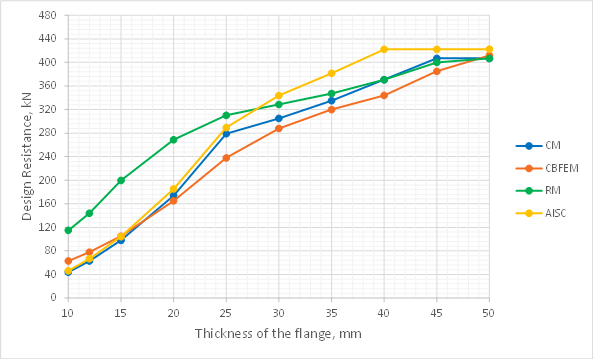Fig. 6 Sensitivity study of flange thickness – adapted from Fig. 5.1.5 of Wald et al. (2020)The size of the finite elements used in IDEA StatiCa can impact the results. To investigate mesh sensitivity, analyses were repeated with four specific maximal element sizes: 2 in., 1 in., 0.5 in., 0.3 in. and compared against previous results using the “default” setting for maximal element size. The minimal element size was equal to 0.3 in. for all analyses except for those with a maximum element size of 0.3 in., in which case the minimal element size was set to 0.2 in. Results are plotted in Fig. 7. Note that the results for maximal element sizes of 2 in. and 1 in. were the same as those for the default maximum element size and are excluded from the plot.Smaller maximal element sizes decrease the maximum load which can be applied to the connection according to IDEA StatiCa. The greatest differences are seen for the thinner plate. As a result, the IDEA StatiCa results with a maximum element size of 0.3 in. compares well to the results from the traditional calculations for the thinnest plates investigated.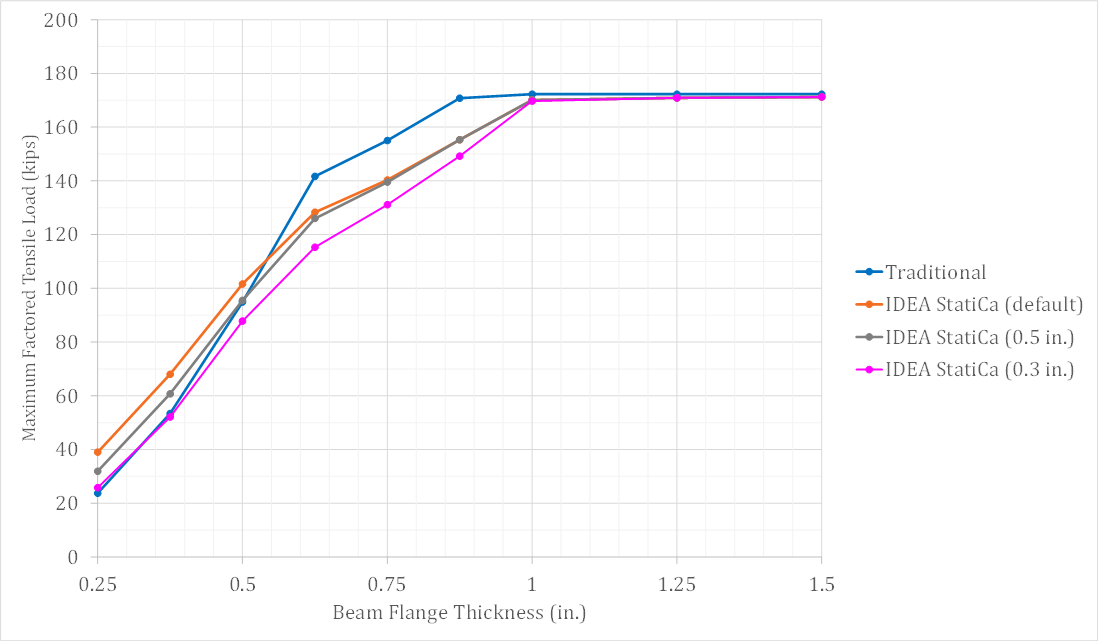Fig. 7 Design strength vs. T-stub flange thickness for bearing-type connections – including mesh sensitivity study Sample files Open in Viewer Download Prying of the beam flangeThe third investigation examines the strength of the beam flange and bolts. The beam flange was varied by selecting different beam sections. Six beam sections were selected for investigation as listed in Tab. 1. To accommodate the greater loads in this investigation, the bolts are Group B (e.g. A490) with threads not excluded from the shear planes; the T-stub flange width was bf = 8.0 in.; the T-stub flange thickness was tf = 1.25 in.; the T-stub web thickness was tw = 0.75 in., and θ = 90°. The connections were not slip-critical. The default mesh settings were used in IDEA StatiCa.Tab. 1 Selected parameters Beam sectiontf (in.)bf (in.) W18×1751.5911.4 W18×1191.0611.3 W18×970.87011.1 W18×760.68011.0 W12×400.5158.01 W10×330.4357.96 The maximum factored tensile load which could be applied to the connection is presented in Fig. 9. As expected, for both the traditional calculation results and the IDEA StatiCa results, the maximum factored tensile load increases with beam flange thickness until a plateau is reached where bending of the T-stub controls. Prying action impacts the strength of each of the connections in this investigation. For the traditional calculations, the guidance of Part 9 of the AISC Manual (2017) was adopted along with the assumed yield-line pattern shown in Fig. 8 (Dowswell 2011). IDEA StatiCa explicitly models the connection using the CBFEM. The pattern of yielding observed from the CBFEM results (Fig. 10) agreed with the assumed yield line used in the traditional calculations. IDEA StatiCa produced conservative results in comparison to the traditional calculations over the range investigated. As before, the IDEA StatiCa results were also compared to a variation of the traditional calculations where Fy was used in lieu of Fu. Use of Fy reduced the strength per the traditional calculations such that it matched closely with the IDEA StatiCa results.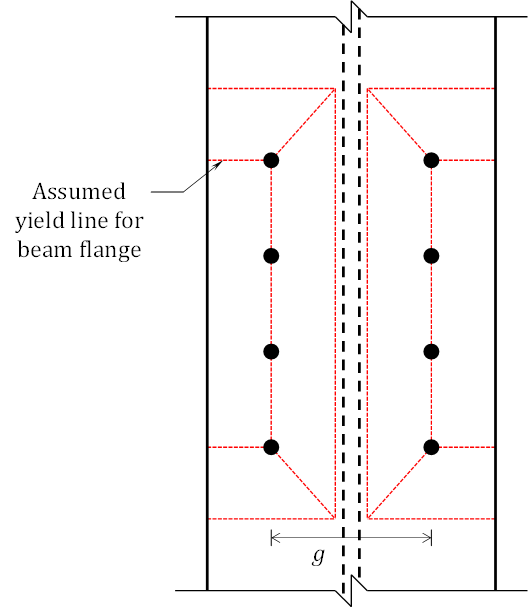Fig. 8 Assumed yield line pattern for beam flange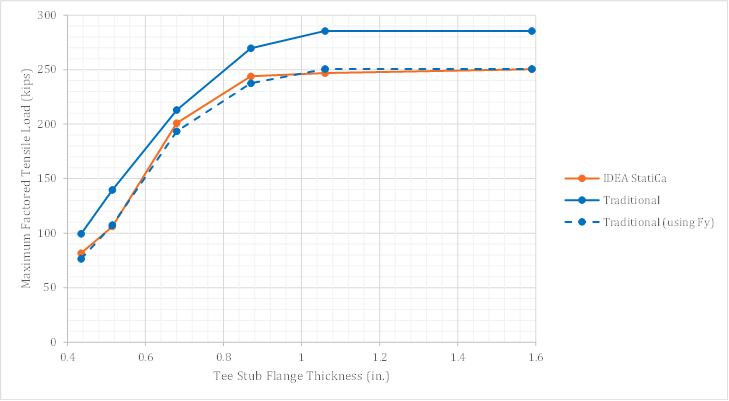Fig. 9 Design strength vs. beam flange thickness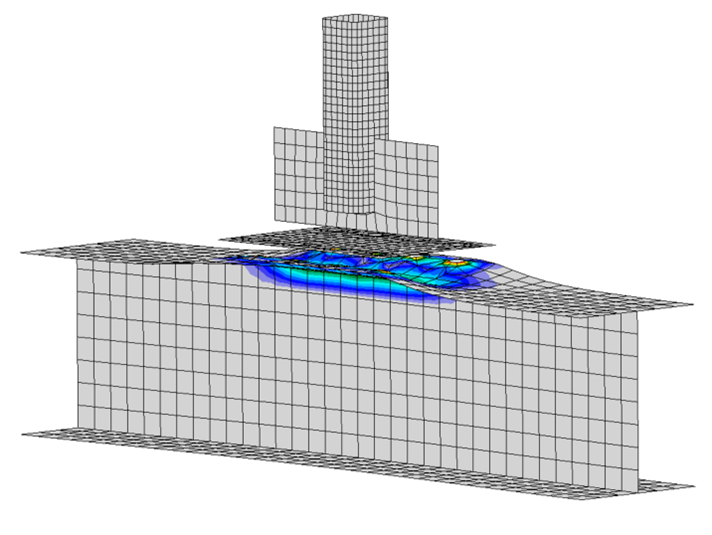Fig. 10 Plastic strain for the connection with a W10×33 beam (deformation scale = 5) Sample files Open in Viewer Download SummaryThis study compared the design of T-stub connections by traditional calculation methods used in US practice and IDEA StatiCa. Key observations from the study include: The available strength obtained from IDEA StatiCa agrees well with the traditional calculations with differences primarily on the conservative side. When evaluating slip-critical connections subjected to combined tension and shear, IDEA StatiCa conservatively considers only the tension in the bolts and not the contact pressure on the faying surfaces (i.e., the prying force) when determining the available strength. Some of the differences in connection strength are due to the equations for prying action presented in Part 9 of the AISC Manual being based on the tensile strength, Fu, while IDEA StatiCa limits the stress to the yield strength, Fy. IDEA StatiCa exhibited greater strength than the traditional calculations for the cases examined with thinner flanges. However, for these cases, there remains a significant margin of safety when compared to the results of a detailed finite element model. Some mesh dependence was observed. For cases where the plastic strain limit controlled, IDEA StatiCa exhibited reduced strengths when the mesh size was set smaller than the default. ReferencesAISC. (2016). Specification for Structural Steel Buildings. American Institute of Steel Construction, Chicago, Illinois.AISC. (2017). Steel Construction Manual, 15th Edition. American Institute of Steel Construction, Chicago, Illinois.AISC. (2019). Steel Construction Manual Design Examples, v15.1. American Institute of Steel Construction, Chicago, Illinois.Dowswell, B. (2011). “A Yield Line Component Method for Bolted Flange Connections.” Engineering Journal, AISC, (2nd Quarter), 93–116.Wald, F., Šabatka, L., Bajer, M., Jehlička, P., Kabeláč, J., Kožich, M., Kuříková, M., and Vild, M. (2020). Component–Based Finite Element Design of Steel Connections. Czech Technical University in Prague.$$

## Related articles

### Bracket Plate Connections (AISC)### Become a certified connection design professional

Ready to master analysis, design, and code-check skills of various steel connections for everyday engineering practice? Our online course can help you